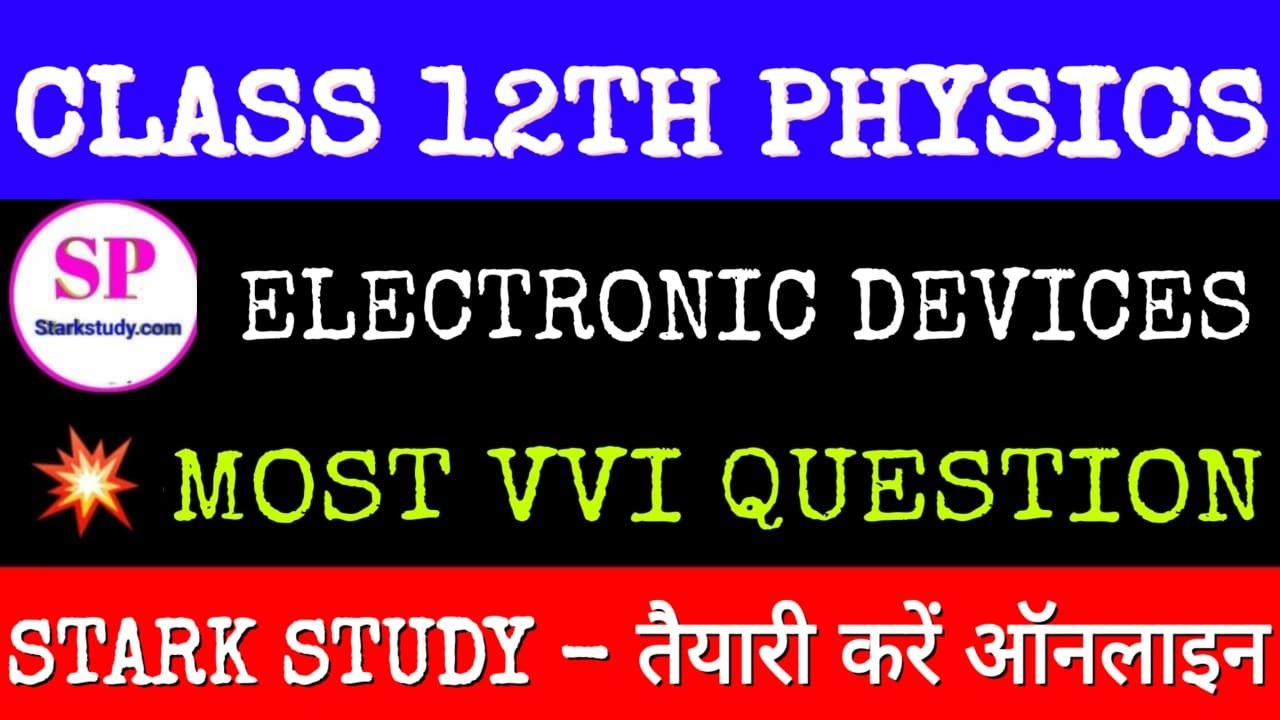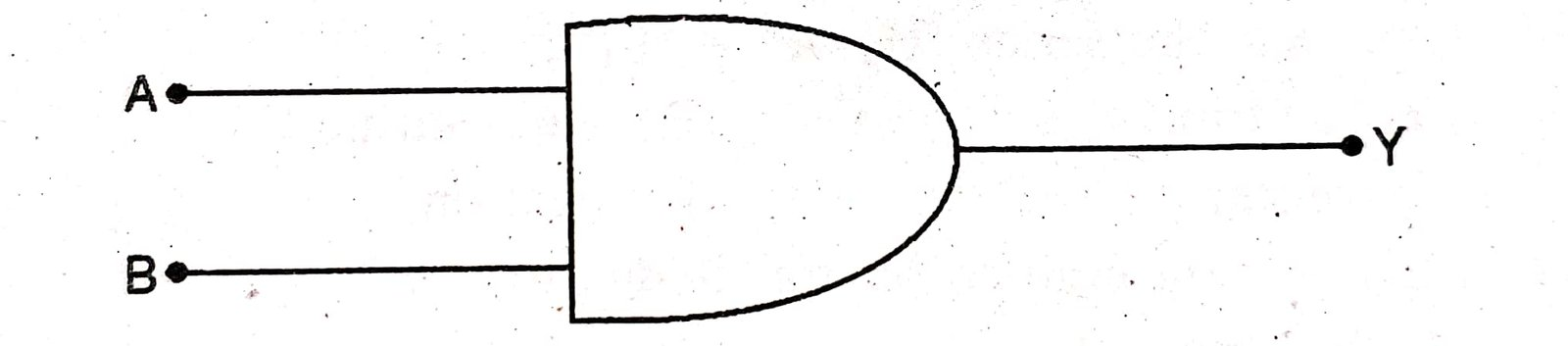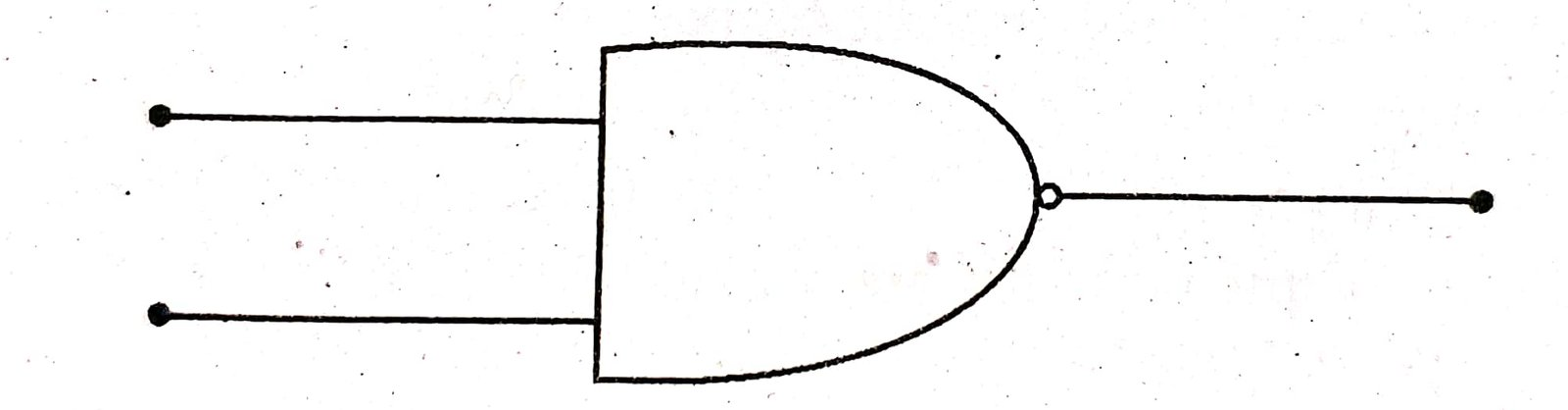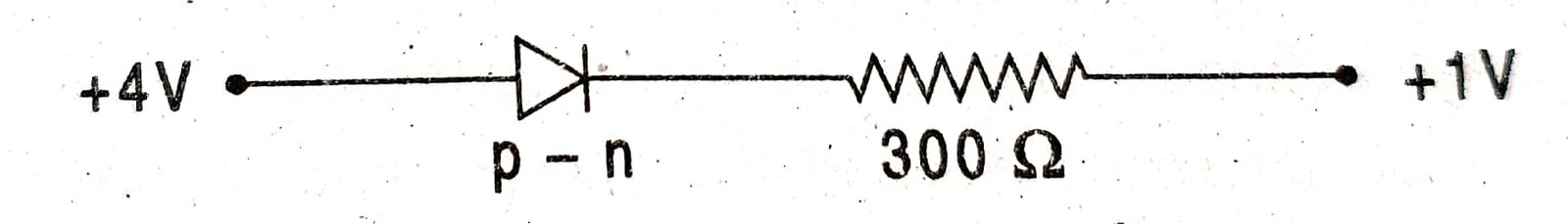Class 12th Physics Most VVI Objective MCQ Question Chapter Wise Electronics Devices

ELECTRONIC DEVICES

1. The impurity doped in germanium to obtain n-type germanium is :

(A) Tetravalent

(B) Trivalent

(C) Pentavalent

(D) None of these

2. In full wave rectification, if input frequency is 50 Hz, then output frequency will be :

(A) 50 Hz

(C) 25 Hz

(B) 100 Hz

(D) 200 Hz

3. Binary of decimal number 25 is :

(A) (1100)2

(B) (1001)2

(C) (11001)2

(D) (11101)2

4 The logic gate shown in figure is :(A) OR

(B) NOR

(C) NAND

(D) AND

5. In ‘n-p-n transistor the relation between emitter current iE base current in and collector current iB is :

(A) iC = iE – i

(B) iB = iE -iC

(C) iE = iC – iB

(D) iB = iE+ iC

6. Main gate (Basic gate) is :

(A) AND, OR

(B) NAND, NOR

(C) OR, NOT

(D) AND, OR, NOT

7. The given symbol represent the Gate :(A) AND

(B) OR

(C) NAND

(D) NOR

8. Boolean algebra is essentially based on :

(B) truth

(A) number

(D) symbol

(C) logic

9. In the circuit given below the value of the current is :(A) 0 A

(B) 10-2

(C) 102

(D) 10-3 A

10. To make a P-type semiconductor Germanium is doped with :

(A) Gallium

(B) Boron

(C) Aluminium

(D) Phosphorus

11. For Binary number 10101, the number in decimal representation will be :

(A) 31

(C) 11

(B) 21

(D) 3

12. With the increases of temperature the resistivity of semiconductor :

(A) increases

(B) decreases

(C) remains constant

(D) becomes zero

13. Who designed the transistor ?

(A) Flemming

(B) Shockley

(C) Bravis

(D) Edison

14. The Boolean expression for NOR gate is :

(A)= Y

(B) A + B = Y

(C) A . B = Y

(D)= Y

15. The majority current-carrier in p-type semiconductor is :

(A) electron

(B) hole

(C) photon

(D) proton

16. Boolean expression of OR gate is :

(A) A + B = C

(B) A · B = 0

(C) Ā= A

(D) C =17. Diode is used as :

(A) An amplifier

(C) A modulator

(B) An oscillator

(D) A rectifier

18. Boolean expression for NAND gate is :

(A)= Y

(B)= Y

(C) A • B = Y

(D) A + B = Y

19. NOT gate in realised by :

(A) n-p-n transistor in common emitter connection

(B) n-p-n transistor in common base connection

(C) p-n-p transistor in common emitter connection

(D) p-n-p transistor in common base connection

20. The decimal number 27 can be written in binary number as :

(A) 110011

(B) 10111

(C) 11001

(D) 10011

21. A semiconductor is cooled from T, K to T, K, then its resistance will :

(A) increase

(B) decrease

(C) remain constant

(D) first decrease then increase

22. If the current constant for a transistor are α & B then : .

(A) αβ = 1

(B) β > 1, α < 1

(C) α = B

(D) β < 1, α > 1

Physics ka Objective Question, 12th Physics Objective Questions and Answers pdf 2021, 12th physics objective questions and answers in Hindi 2021, physics objective questions for 12th Bihar board, 12th physics objective questions and answers in Hindi 2021 pdf, physics 12th objective 2020 pdf, physics 12th objective 2021, physics important questions class 12 State Board 2021, 12th Board Exam Physics Chapter 2 Electric Potential And Capacitance Objective Question, Class 12th Physics Chapter 3 Current Electricity Important Objective Question,12th Board Exam Physics Chapter 4 Moving Charges & Magnetism Objective Question, Class 12th Physics Chapter 6 Electromagnetic Induction VVI Objective Question, Class 12th Physics Chapter 8 Electro-Magnetic Waves VVI Objective Question, Class 12th Physics Chapter 10 Wave Optics VVI Objective Question, 12th Exam 2021 Question paper Download, Bihar Board 12th Question Paper 2021

 10TH 12TH MOBILE APP Class 10th CLICK Class 12th CLICK 10th Mobile App CLICK 12th Mobile App CLICK
 12TH BOARD SCIENCE STREAM Physics CLICK Chemistry CLICK Biology CLICK Mathematics Hindi 100 English 100 Hindi 50 English 50 CLICK

Class 12th Physics Chapter Wise Electric Charges & Fields VVI Objective Question, 12th physics MCQ with answers 2021, 12th ka physics objective,12 class objective question answer, class 12  Hindi objective question, physics objective book in Hindi pdf, class 11 physics objective questions in Hindi, Physics 12th objective answer 2021, physics objective questions for 12th pdf, Class 12th Physics Chapter 3 Current Electricity Important Objective Question, 12th physics objective question test, physics 12th objective optics, VVI question physics class 12 VVI objective, Class 12th Physics Chapter 5 Magnetism & Matter VVI Objective Question,  Class 10th Objective,

Class 12th Physics Most VVI Objective Alternating Current Chapter, BSEB Inter Exam 2021 Important Guess Question Paper, 12th physics Paper, Class 12th Physics Objective Question Nuclei Chapter on New Pattern, Class 12th Physics Most VVI Objective MCQ Question Chapter Wise Electronics Devices

## 1 thought on “”

1. Khushi choudhary says:

Sir please subjective bhi de dijiye chemistry or physics ka